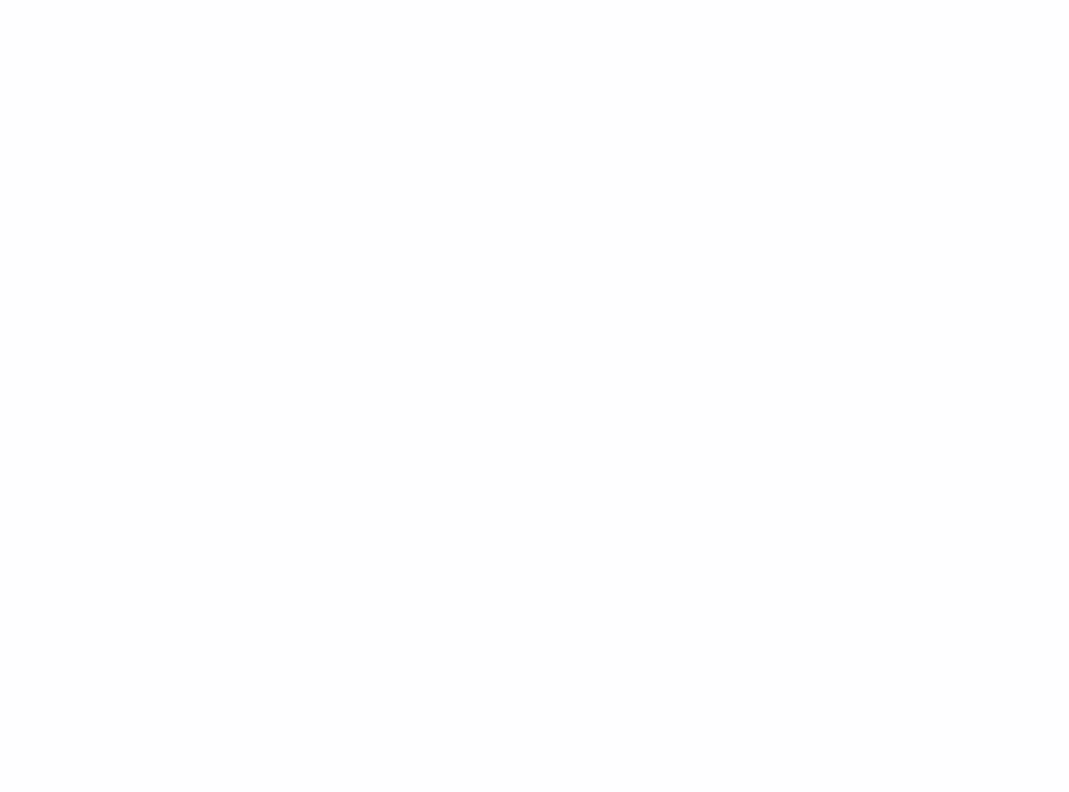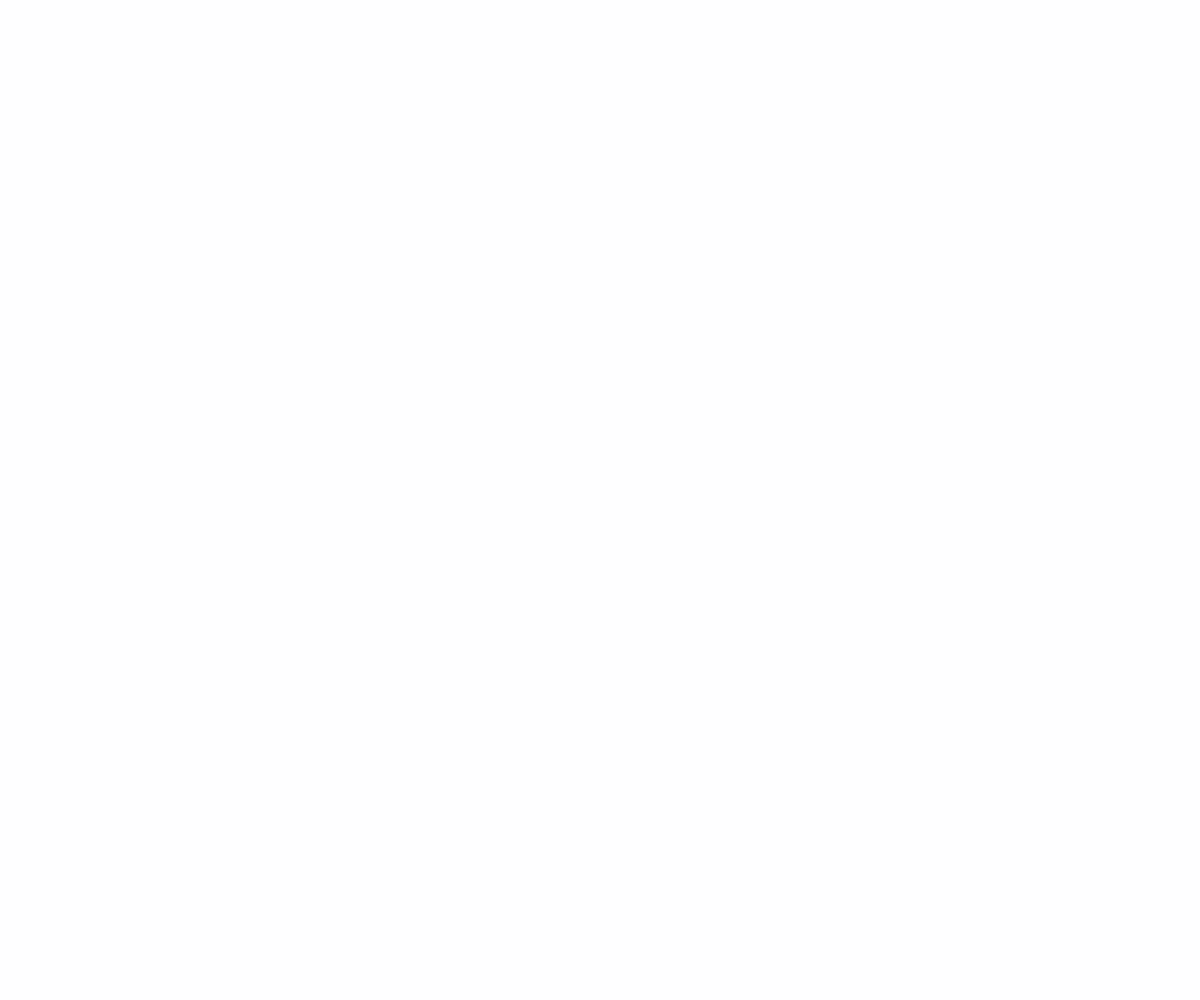# Create animated histograms with RAnimated histograms that can be embedded directly into a publication on any site are becoming increasingly popular. They display the dynamics of changes in any characteristics for a certain time and they do it clearly. Let's see how to create them with R and universal packages.

Skillbox recommends: Practical course "Python-developer from scratch."

We remind: for all readers of "Habra" – a discount of 10,000 rubles when writing to any Skillbox course using the promo code "Habr".

### Packages

We need packages in R:

• ggplot2
• gganimate

These two are extremely necessary. In addition, tidyverse, janitor and scales are required for data management, array cleaning and formatting, respectively.

### Data

The original data set, which we will use in this project, is downloaded from the World Bank website. Here they are – WorldBank Data. The same data, if you need it in finished form, can be downloaded from the project folder.

What is this information? The sample contains the GDP value of most countries over several years (from 2000 to 2017).

### Data processing

We will use the code below to prepare the necessary data format. We clear the column names, turn the numbers into a number format, and convert the data using the function gather (). Everything that is received is saved in gdp_tidy.csv for further use.

``````library (tidyverse)
library (janitor)

gdp <- read_csv ("./ data / GDP_Data.csv")

#select required columns

gdp <- gdp %>% select (3:15)

#filter only country rows

gdp <- gdp[1:217,]

gdp_tidy <- gdp %>%
mutate_at (vars (contains ("YR")), as.numeric)%>%
gather (year, value, 3:13)%>%
janitor :: clean_names ()%>%
mutate (year = as.numeric (stringr :: str_sub (year, 1,4)))

write_csv (gdp_tidy, "./ data / gdp_tidy.csv")``````

### Animated histograms

Their creation requires two stages:

• Build a complete set of current histograms using ggplot2.
• Animate static histograms with desired parameters using gganimate.

The final step is to render the animation in the desired format, including GIF or MP4.

• library (tidyverse)
• library (gganimate)

### Data management

In this step, you need to filter the data to get the top 10 countries of each year. Add a few columns that allow you to display the legend for the histogram.

``````gdp_tidy <- read_csv ("./ data / gdp_tidy.csv")

gdp_formatted <- gdp_tidy %>%
group_by (year)%>%
# The * 1 makes it possible to have non-integer ranks while sliding
mutate (rank = rank (-value),
Value_rel = value / value[rank==1],
Value_lbl = paste0 ("", round (value / 1e9)))%>%
group_by (country_name)%>%
filter (rank <=10) %>%
ungroup ()``````

### Construction of static histograms

Now that we have the data package in the right format, we start drawing static histograms. Basic information – the top 10 countries with the maximum GDP for the selected time interval. We build graphs for each year.

``````staticplot = ggplot (gdp_formatted, aes (rank, group = country_name,
fill = as.factor (country_name), color = as.factor (country_name))) +
geom_tile (aes (y = value / 2,
height = value,
width = 0.9), alpha = 0.8, color = NA) +
geom_text (aes (y = 0, label = paste (country_name, "")), vjust = 0.2, hjust = 1) +
geom_text (aes (y = value, label = Value_lbl, hjust = 0)) +
coord_flip (clip = "off", expand = FALSE) +
scale_y_continuous (labels = scales :: comma) +
scale_x_reverse () +
guides (color = FALSE, fill = FALSE) +
theme (axis.line = element_blank (),
axis.text.x = element_blank (),
axis.text.y = element_blank (),
axis.ticks = element_blank (),
axis.title.x = element_blank (),
axis.title.y = element_blank (),
legend.position = "none",
panel.background = element_blank (),
panel.border = element_blank (),
panel.grid.major = element_blank (),
panel.grid.minor = element_blank (),
panel.grid.major.x = element_line (size = .1, color = "gray"),
panel.grid.minor.x = element_line (size = .1, color = "gray"),
plot.title = element_text (size = 25, hjust = 0.5, face = "bold", color = "gray", vjust = -1),
plot.subtitle = element_text (size = 18, hjust = 0.5, face = "italic", color = "gray"),
plot.caption = element_text (size = 8, hjust = 0.5, face = "italic", color = "gray"),
plot.background = element_blank (),
plot.margin = margin (2,2, 2, 4, "cm"))``````

Building graphics using ggplot2 is quite simple. As you can see in the code section above, there are a few key points with the theme () function. They are needed so that all elements are animated without problems. Some of them can not be displayed if necessary. Example: only vertical grid lines and legends are drawn, but the headers of the axes and several more components are removed from the section.

### Animation

The key function here is transition_states (), it sticks together individual static graphs. view_follow () is used to draw grid lines.

``````anim = staticplot + transition_states (year, transition_length = 4, state_length = 1) +
labs (title = 'GDP per Year: {closest_state}',
subtitle = "Top 10 Countries",
caption = "GDP in Billions USD | Data Source: World Bank Data")``````

### Rendering

After the animation is created and saved in the anim object, it is time to render it using the animate () function. The renderer used in animate () may vary depending on the type of output file required.

Gif

``````# For GIF

animate (anim, 200, fps = 20, width = 1200, height = 1000,
renderer = gifski_renderer ("gganim.gif"))
``````

Mp4

``````# For MP4

animate (anim, 200, fps = 20, width = 1200, height = 1000,
renderer = ffmpeg_renderer ()) -> for_mp4

anim_save ("animation.mp4", animation = for_mp4)``````

### ResultAs you can see, nothing complicated. The whole project is available in my GitHub, you can use it as you see fit.

Skillbox recommends:

• Two-year practical course "I am a web developer PRO".
• Online course "From # developer from scratch."
• Practical annual course "PHP developer from 0 to PRO".